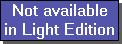The SDL Component Suite is an industry leading collection of components supporting scientific and engineering computing. Please visit the SDL Web site for more information....## ExpressionUnit: SDL_math2 Class: Declaration: property Expression: string;

The property Expression contains the mathematical or logical expression to be evaluated. The expression is not case sensitive, however you should be careful to avoid improper mixing of boolean and arithmetic subexpressions, for example:
(a>5) and (b=0) yields a boolean result, while
(a+5) and (b=0) yields an integer value (the and operator is used as a bitwise and)

You may use any number of user-defined variables, provided that the variable names are not equal to any of the reserved function names (see below). A user-defined variable always starts with a letter and may consist of any number of digits and letters and the underscore character ('_'). See Evaluate for further details on variables.

The expression may use the following pre-defined constants, operators, and functions:

 --- Constants --- true logical true (or -1, if used as number) false logical false (or 0, if used as number) pi the number Pi (3.14159...) --- References --- ° currently referenced cell; this symbol indicates the value of the currently referenced cell when iterating through a range of cells. It is treated as a (predefined) variable. Thus the user has to supply the actual value in the OnVarRequest event. --- Arithmetic Operators --- + sum: x+y - difference: x-y * product: x*y / division: x/y # modulo: round(x) mod round(y) ^ power: exponentiation x^y , x>0, y..any real values --- Logic Operators --- > greater than >= greater than or equal = equal >< not equal < less than <= less than or equal and boolean or bitwise and not boolean or bitwise not or boolean or bitwise or xor boolean or bitwise exclusive or --- Functions --- abs absolute value: abs(x), x..any real value arccos inverse cosine: arccos(x), x..angle in radians arcsin inverse sine: arcsin(x), x..angle in radians arctan inverse tangens: arctan(x), x..angle in radians cos cosine: cos(x), x..angle in radians exp exponential function: exp(x) frac fraction: frac(x) = x - int(x) gauss gauss creates normally distributed random numbers with zero mean and unit standard deviation int round towards zero: int(x) lg decadic logarithm: lg(x) ln natural logarithm: ln(x) mean returns the mean of a list of variables: mean(list), with list containing a list of variables reparated by commas;(1) a range of numbered variables may be abbreviated by the ':' sign (i.e. "xx8:11" expands to "xx8, xx9, xx10, xx11"). nddens density of the standard normal distribution: nddens(x) ndint integral of the standard normal distribution from -infinity to x: ndint(x) ndquant quantile of the standard normal distribution for a probability x: ndquant(x) rand uniformly distributed random numbers: random(x), x..amplitude of noise (mean = 0.0) round round to the nearest integer: round(x) sign sign of x: sign(x) sin sinus: sin(x) sqr square: sqr(x) sqrt square root: sqrt(x) sum returns the sum of a list of variables: sum(list), with list containing a list of variables reparated by commas;(1) a range of numbered variables may be abbreviated by the ':' sign (i.e. "zz1:3" expands to "zz1, zz2, zz3"). tan tangens: tan(x) var returns the variance of a list of variables: var(list), with list containing a list of variables reparated by commas;(1) a range of numbered variables may be abbreviated by the ':' sign (i.e. "xx8:10,aux4,y1:3" expands to "xx8, xx9, xx10, aux4, y1, y2, y3").

 (1) The maximum number of list elements may not exceed 2000. However the exact limit depends on the structure of the formula, so the maximum number is slightly lower (most often between 1980 and 1999).

Last Update: 2014-Jun-07